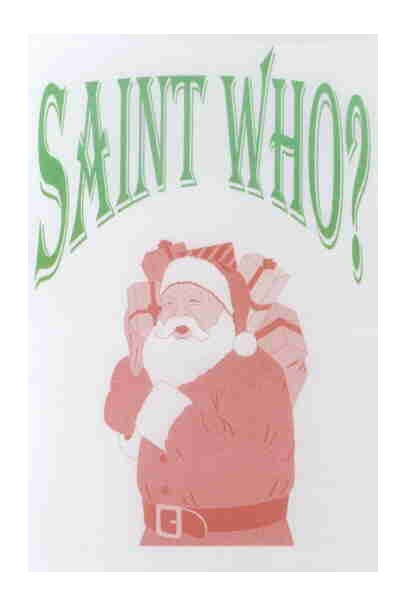WINNERS CHRISTMAS PROBLEM

The main prize goes to doctor Ronald A Kortram, maths professor at the mathematics and science department of the Nijmegen Catholic University.
I am very grateful for the friendliness which made him participate in the competition.
In his solution he begins with two points B en C with equal y-coordinates, which simplifies the calculations.
Furthermore, he elegantly applies a theorem of Ptolemy to prove the correctness of his outcome.
His reasoning is very precise and clear.
His solution is given below in full extent.

Secondly, I was glad to receive a fine solution by doctor Jos KM Jansen, maths professor at the mathematics and computer science department of the Eindhoven University of Technology.
I am grateful for the friendliness which made him participate in the competition.
He showed me fine applications of geometry theorems before, for example the line of Euler and the theorem of Stewart.
He finds numerical answers using the computer algebra program Mathematica, and thereafter the exact outcome, using the point of Torricelli.
His proof is a bit short: he says that by symmetry the extremes must here appear in triangles with at least two equal sides.
For appreciation of all his contributions, I give him a prize as well.

In the junior-A category, the prize goes to Martien Luijcks, master of pedagogics and social worker at Haarlem.
He gives a visual solution and finds the exact positions for the maximum, and an approximation of the right positions for the minimum. He also finds reasonable approximations for the values of the extremes (3 and 5).
He uses the words 'center of mass', but in such a way that this does not imply disqualification for category A. By the way, Martien, the mathematical triangle centroid is not involved in the correct solution.

In category B, the prize goes to mr Armand Schneider from Aachen. I let him speak for himself:

I work with Cabri. I have drawn a circle with a radius of 5 cm. I don't know how to change the unit length, so lengths are 5 times too large and areas are 25 times too large.
I make a triangle ABC with A,B,C at the circle (A at 12 o'clock, B at 9 o'clock, C at 3 o'clock). The area of this triangle is 25 cm2.
I set a point P on the circle and draw the straight line segments PA,PB,PC. I let Cabri measure the lengths of these line segments. When I move P along the circle, the distance sum PA+PB+PC seems to be maximal when P is at 6 o'clock. The sum is then 24.15 cm.
Now I move B along the circle in the direction of 6 o'clock, and C in the direction of 12 o'clock, and keep the area of the triangle (as measured by Cabri) equal to 25 cm2.
Then I have to move P a bit from 6 o'clock in the direction of 3 o'clock, to keep the distance sum maximal, but the maximum is now 24.13 cm.
I repeat this procedure, moving P a bit further, and now I get maximal sum 23.93 cm.
So the overall answer seems to be 24.15. I divide by 5 and get 4.83. The exact answer probably is 2+root(2)+root(2).

To determine the minimal distance sum, I start again with the rectangular triangle with points A,B,C at 12,9 and 3 o'clock resp.
I set a point P inside the triangle, draw the line segments PA,PB,PC, and have Cabri measure the lengths of these line segments. When I move P around, the sum seems to be minimal when PB=PC and P is a bit nearer to A than to BC. The sum is then 13.67 cm.
When I move B along the circle in the direction of 6 o'clock, and C in the direction of 12 o'clock, keepig the area of triangle ABC at 25 cm2, the new minimal value is 13.73 cm.
So it seems that the minimal distance sum in all possible triangles is about 13.67 cm, and that this minimal distance sum appears in the rectangular triangle with points A,B,C at 12, 9 and 3 o'clock.
I divide by 5 and get 2.73. The exact answer could be root(3)+1.

Well done, Armand.

The solution of main prize winner dr Kortram:  click here.